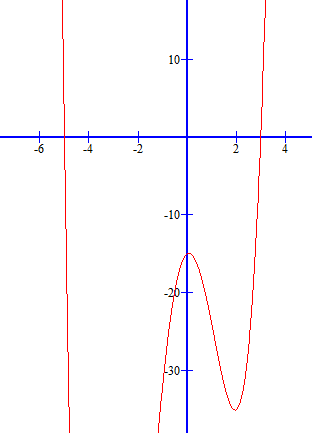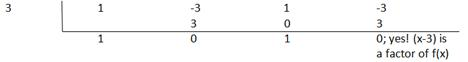### Sample Problem

Find all the rational zeros of the function p(x)=x4+2x3-14x2+2x-15 (order from least to greatest)

x= ,

#### Solution

The possible rational zeros (by the Rational Zero Test) are as follows:

x=±3, ±5, ±1, ±15

Take a look at the graph of this function to see which x values you can rule out and test the x values that make sense using synthetic division:Looking at this graph, we know that the only rational zeros that are possible are x=-5 and x=3.The complete factorization of x4+2x3-14x2+2x-15= (x+5)(x-3)(x2+1)

[note: the solutions to x2+1 are not real, therefore are not included in the “rational zeros” of this function]